• 本文将要介绍的内容很简单，就是如何根据一组非线性相关的向量来计算一组标准正交基。但是与其他文章不同的是，本文将以一种非常直观的思路来，顺理成章的推导出如何计算标准正交基。 首先我们假设有一组非线性相关...
UTF8gbsn
本文将要介绍的内容很简单，就是如何根据一组非线性相关的向量来计算一组标准正交基。但是与其他文章不同的是，本文将以一种非常直观的思路来，顺理成章的推导出如何计算标准正交基。
首先我们假设有一组非线性相关的基

v

1

,

v

2

,

.

.

.

,

v

n

∈

V

v_1,v_2,...,v_n\in V

，我们如何根据

v

1

,

v

2

,

.

.

.

,

v

n

v_1,v_2,...,v_n

来计算

V

V

空间的一组标准正交基？
把

v

1

v_1

看做一个一维空间的基，那么自然计算一个标准基的算法为

e

1

=

v

1

∣

v

1

∣

e_1=\frac{v_1}{|v_1|}

现在我们已经有一个标准基向量

e

1

e_1

,那么我们新加入

v

2

v_2

，他们会行成一个平面。假设

e

1

,

e

2

e_1,e_2

就是这个平面的一组标准正交基，那么

v

2

v_2

一定可以表示为，

v

2

=

∣

v

2

∣

[

c

o

s

(

α

)

e

1

+

s

i

n

(

α

)

e

2

]

v_2=|v_2|[cos(\alpha)e_1+sin(\alpha)e_2]

,接下来反求

e

2

e_2

就可以了。 故而下面的等式成立.这个是可以通过简单的几何画图直观上就可以看出来的。

v

2

∣

v

2

∣

−

c

o

s

(

α

)

e

1

=

s

i

n

(

α

)

e

2

,

c

o

s

(

α

)

=

v

2

⋅

e

1

∣

v

2

∣

\frac{v_2}{|v_2|}-cos(\alpha)e_1=sin(\alpha)e_2,cos(\alpha)=\frac{v_2\cdot e_1}{|v_2|}

E

2

=

v

2

−

(

v

2

⋅

e

1

)

e

1

⇒

e

2

=

E

2

∣

E

2

∣

E_2 = v_2-(v_2\cdot e_1)e_1\Rightarrow e_2 = \frac{E_2}{|E_2|}

同理在

e

1

,

e

2

e_1,e_2

所长成的二维空间上，加入新的

v

3

v_3

，可以张成一个三维空间。我们现在假定

e

1

,

e

2

,

e

3

e_1,e_2,e_3

张成了一个空间。那么现在我们有

v

3

∣

v

3

∣

=

c

o

s

(

α

)

e

1

+

c

o

s

(

β

)

e

2

+

c

o

s

(

γ

)

e

3

\frac{v_3}{|v_3|}=cos(\alpha)e_1+cos(\beta)e_2+cos(\gamma)e_3

, 其中

α

,

β

,

γ

\alpha,\beta,\gamma

分别是

v

3

v_3

与三个基向量之间的夹角。

E

3

=

v

3

−

(

v

3

⋅

e

2

)

e

2

−

(

v

3

⋅

e

1

)

e

1

⇒

e

3

=

E

3

∣

E

3

∣

E_3=v_3-(v_3\cdot e_2)e_2-(v_3\cdot e_1)e_1\Rightarrow e_3=\frac{E_3}{|E_3|}

同理我们可以求出

e

n

e_n

E

n

=

v

n

−

∑

k

=

1

n

−

1

(

v

n

⋅

e

k

)

e

k

⇒

e

n

=

E

n

∣

E

n

∣

E_n=v_n-\sum_{k=1}^{n-1}(v_n\cdot e_k)e_k\Rightarrow e_n=\frac{E_n}{|E_n|}

来总结一下，也就是每一次假设引入一个标准基向量

e

k

e_k

，和前面求出来的标准基向量

e

1

,

e

2

,

.

.

.

,

e

k

e_1,e_2,...,e_k

来组成一个空间。这一组新的标准正交基可以组合为新加入的

v

k

v_k

,饭后根据

v

k

v_k

可

e

1

,

e

2

,

.

.

.

,

e

k

e_1,e_2,...,e_k

之间的夹角关系求出

e

k

e_k

,因为夹角很好求，是

v

k

⋅

e

j

∣

v

k

∣

,

j

∈

(

1

,

2

,

.

.

.

,

k

)

\frac{v_k\cdot e_j}{|v_k|},j\in (1,2,...,k)

,所以很好求出

e

k

e_k

，其实这里面只是利用了一个向量加法而已。
展开全文向量
• ## 空间的基，自然基，标准正交基

千次阅读 多人点赞 2018-12-17 10:43:31
误区：总以为二维平面是垂直的x轴和y轴 总以为三维空间的是垂直的x轴，y轴和z轴 解析： 以二维空间为例，不光只有垂直的两个向量才能表示整个空间。 如上图所示，垂直的两个向量能表示整个平面自不必多...
误区：总以为二维平面的基是垂直的x轴和y轴 总以为三维空间的基是垂直的x轴，y轴和z轴
解析：
以二维空间为例，不光只有垂直的两个向量才能表示整个空间。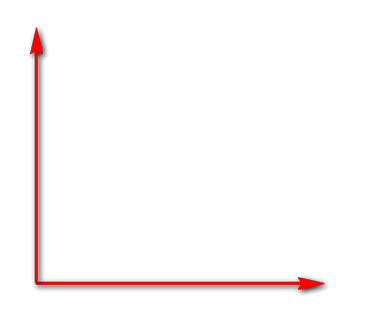如上图所示，垂直的两个向量能表示整个平面自不必多说。
下图中两个不垂直的向量，也可以表示整个平面。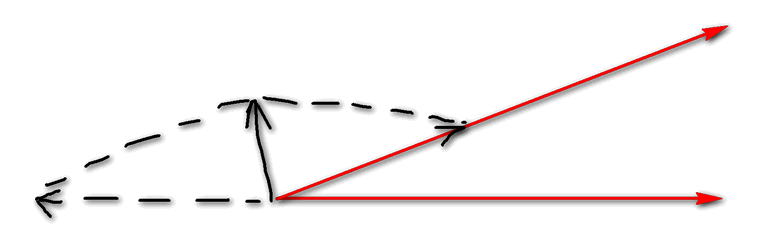综上，二维平面中，凡是不平行的两个向量都称为“基”
推理，三维空间中，凡是不在一个平面中的3个向量都可以表示整个三维空间，作为3维空间的“基”。
引申，高维空间的“基”，一定不会同时存在于低纬空间中。如果同时存在于低纬空间，则线性相关，不能称为“基”。
举例：三维空间的“基”不能同时存在于二维空间（平面）中，因为平面由两个不平行的向量就可以表示，如果第三个向量如果也在这个平面当中，完全可以由另外两个向量表示出来（线性相关），那么这样的3个向量其实到最后仍然只能表示一个平面，无法表示3维空间。
综上：两个不平行的向量就是二维平面的“基”，3个不在同一平面中的向量就是3维平面的“基”。即：n维空间可以用n个线性不相关的向量表示。
把向量装入矩阵，就成为求矩阵的“秩”，“秩”为几就可以表示几维的空间。
假设5个向量，“秩”为3，那么这5个向量只能表示3维空间。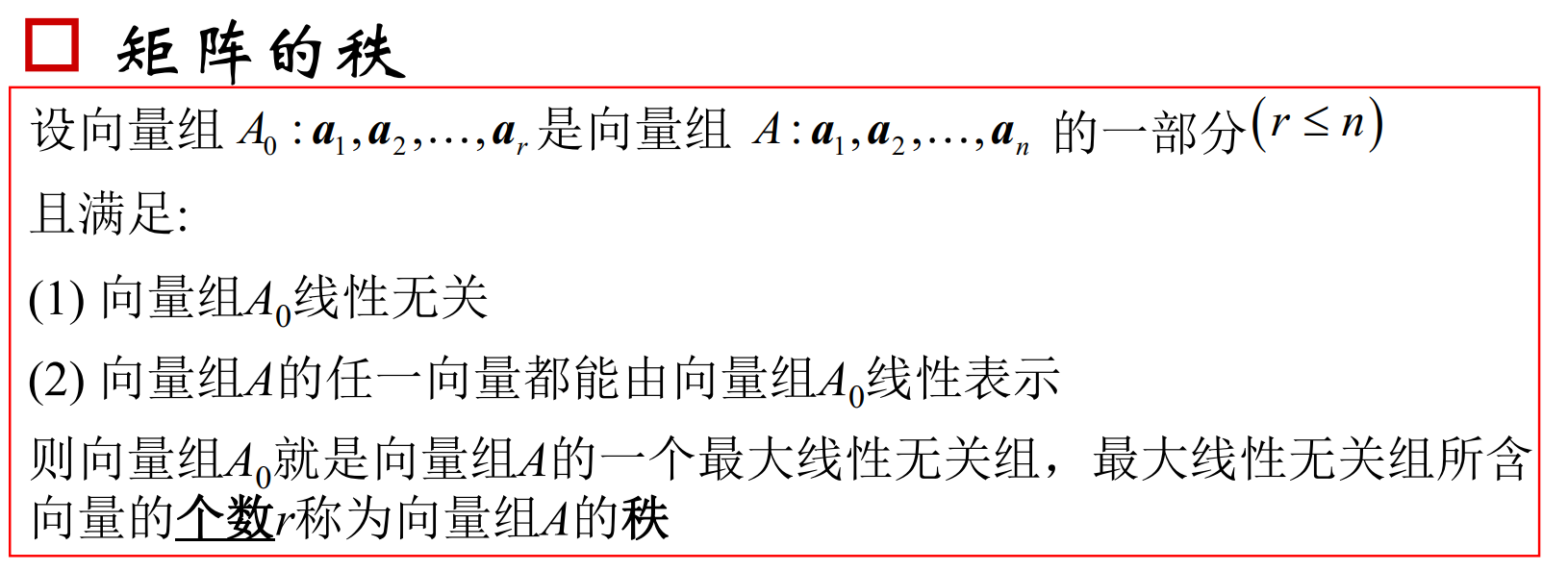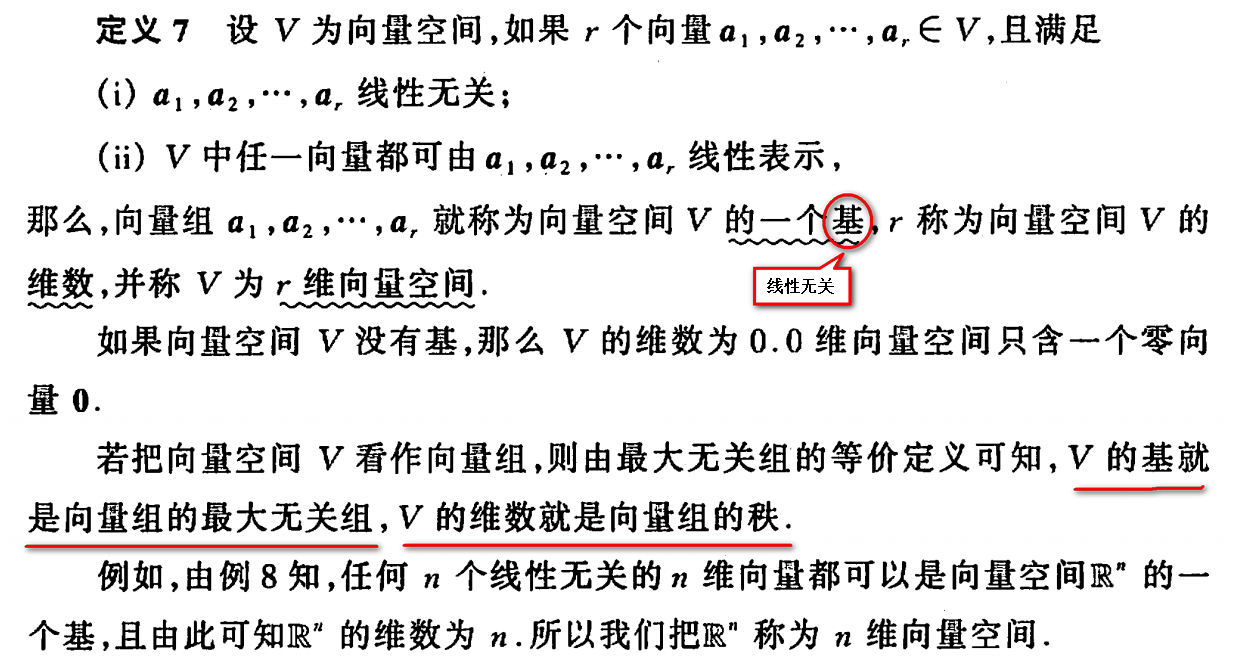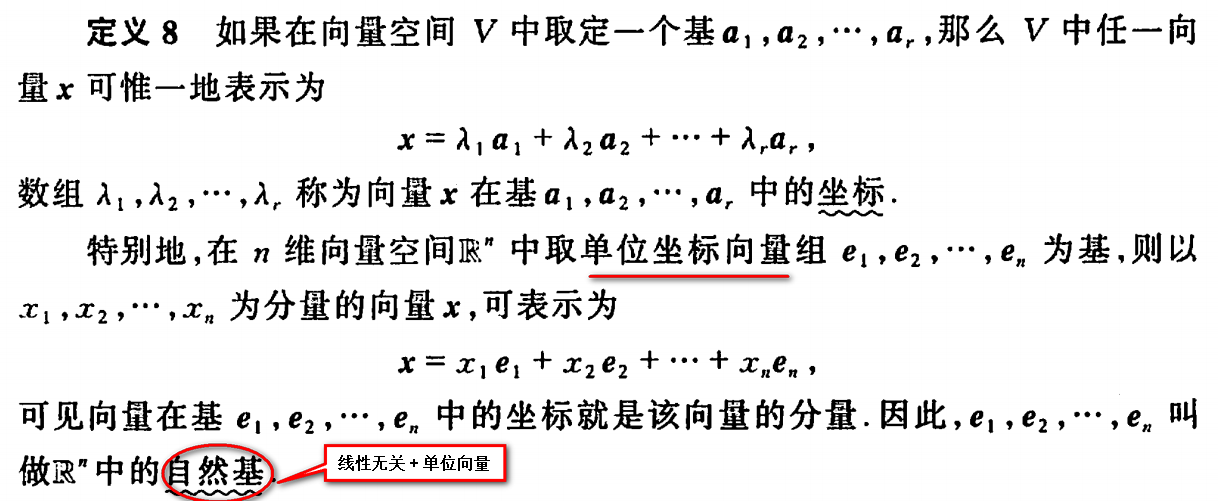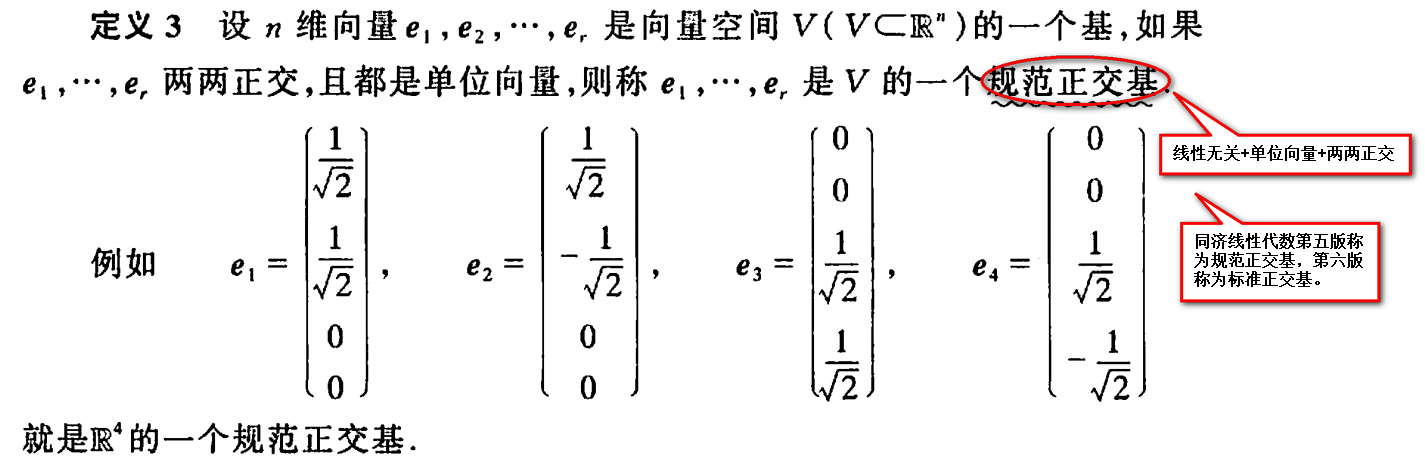展开全文• 对于一个正交基，每个向量和其他所有向量垂直，坐标轴就是互相正交的。我们还可以进一步改善：每个向量除以它的长度得到单位向量，这样的话正交基变成了标准正交基：16、如果 qTiqj={01i≠j,给出正交性i=j,给出归一...
对于一个正交基，每个向量和其他所有向量垂直，坐标轴就是互相正交的。我们还可以进一步改善：每个向量除以它的长度得到单位向量，这样的话正交基变成了标准正交基：
16、如果

qTiqj={01i≠j,给出正交性i=j,给出归一性

q_i^Tq_j=
\begin{cases}
0&i\neq j,\text{给出正交性}\\
1&i=j,\text{给出归一性}
\end{cases}

那么

q1,…,qn
$q_1,\ldots,q_n$就是是标准正交基，由标准正交列组成的矩阵叫做

Q
$Q$。

最重要的例子是标准基，对于x−y$x-y$平面，最熟悉的

e1=(1,0)，e2=(0,1)
$e_1=(1,0)，e_2=(0,1)$水平和竖直方向都是垂直的，

Q
$Q$是2×2$2\times 2$的单位矩阵。在

n
$n$为空间里标准基e1,…,en$e_1,\ldots,e_n$由

Q=I
$Q=I$的列组成：

e1=⎡⎣⎢⎢⎢⎢⎢⎢⎢100⋮0⎤⎦⎥⎥⎥⎥⎥⎥⎥,e2=⎡⎣⎢⎢⎢⎢⎢⎢⎢010⋮0⎤⎦⎥⎥⎥⎥⎥⎥⎥,⋯,en=⎡⎣⎢⎢⎢⎢⎢⎢⎢000⋮1⎤⎦⎥⎥⎥⎥⎥⎥⎥.

e_1=\begin{bmatrix}
1\\0\\0\\\vdots\\0
\end{bmatrix},
e_2=\begin{bmatrix}
0\\1\\0\\\vdots\\0
\end{bmatrix}
,\cdots,
e_n=\begin{bmatrix}
0\\0\\0\\\vdots\\1
\end{bmatrix}.
这不是唯一的正交基！我们在不改变直角的情况下悬着这些轴，依然是正交基，旋转得到的矩阵我们用

Q
$Q$来表示。

如果我们有一个Rn$R^n$的子空间，标准向量

ei
$e_i$可能不在这个子空间，但是子空间肯定有一个正交基，我们可以通过一种简单的方法来构造出来，本质上就是将这些倾斜的轴变成正交的，这就是所谓的格拉姆-施密特正交化(Gram-Schmidt othogonalization)。 接下来，说一些本篇文章的是三个主题：
正交矩阵

Q
$Q$的定义和性质。
Qx=b$Qx=b$的解，其中

Q
$Q$可以是n×n$n\times n$方阵，也可以是长方形矩阵(最小二乘)。格拉姆-施密特方法的具体步骤以及新的矩阵分解

A=QR
$A=QR$。 正交矩阵 17、如果

Q
$Q$(正方行或长方形)的列是正交的，那么QTQ=I$Q^TQ=I$：

⎡⎣⎢⎢⎢⎢⎢−−−qT1qT2⋮qTn−−−⎤⎦⎥⎥⎥⎥⎥⎡⎣⎢|q1||q2|⋯|qn|⎤⎦⎥=⎡⎣⎢⎢⎢10⋅001⋅0⋅⋅⋅⋅00⋅1⎤⎦⎥⎥⎥(1)

\begin{equation}
\begin{bmatrix}
-&q_1^T&-\\
-&q_2^T&-\\
&\vdots&\\
-&q_n^T&-
\end{bmatrix}
\begin{bmatrix}
|&|&&|\\
q_1&q_2&\cdots&q_n\\
|&|&&|
\end{bmatrix}
=\begin{bmatrix}
1&0&\cdot&0\\
0&1&\cdot&0\\
\cdot&\cdot&\cdot&\cdot\\
0&0&\cdot&1
\end{bmatrix}\tag1
\end{equation} 正交矩阵就是列是单位正交的方阵，它的转置等于它的逆，即

QT=Q−1
$Q^T=Q^{-1}$。 当

QT
$Q^T$的

i
$i$行乘以Q$Q$的

j
$j$列时，结果为零qTiqj=0$q_i^Tq_j=0$，而对角线上

i=j
$i=j$，得到

qTiqi=1
$q_i^Tq_i=1$，也就是长度为1的单位矩阵归一化。 注意即便

Q
$Q$是长方形矩阵，QTQ=I$Q^TQ=I$依然成立，但是

QT
$Q^T$仅仅是左逆。 例1：

Q=[cosθsinθ−sinθcosθ],QT=Q−1=[cosθ−sinθsinθcosθ]

Q=\begin{bmatrix}
\cos\theta&-\sin\theta\\
\sin\theta&\cos\theta
Q^T=Q^{-1}=
\begin{bmatrix}
\cos\theta&\sin\theta\\
-\sin\theta&\cos\theta
\end{bmatrix}

Q
$Q$将每个向量旋转θ$\theta$度，而

QT
$Q^T$旋转

−θ
$-\theta$度，相当于有回到原点了。这些列明显都是正交的，因为

sin2θ+cos2θ=1
$\sin^2\theta+\cos^2\theta=1$。 例2：置换矩阵

P
$P$都是正交矩阵，它的列都是单位向量，而且正交。因为每一列在不同的位置有一个1，它的转置也等于它的逆：
P=⎡⎣⎢001100010⎤⎦⎥那么P−1=PT=⎡⎣⎢001100010⎤⎦⎥
P=\begin{bmatrix}
0&1&0\\0&0&1\\1&0&0
\end{bmatrix}\text{那么}
0&1&0\\0&0&1\\1&0&0
\end{bmatrix}
反对角线

P
$P$上的元素P13=P22=P31=I$P_{13}=P_{22}=P_{31}=I$将

x−y−z
$x-y-z$轴变成了

z−y−x
$z-y-x$轴——也就是右手系统变成了左手系统，所以如我们说每个正交矩阵

Q
$Q$表示一个旋转的话就不是很准确，因为反射也能做到:
P=
P=\begin{bmatrix}
0&1\\1&0
\end{bmatrix}

将点

(x,y)
$(x,y)$反射成

(y,x)
$(y,x)$，它的镜像是

45∘
$45^{\circ}$斜线。从几何上来看，正交矩阵

Q
$Q$是旋转矩阵和反射矩阵的乘积。

有一个性质是旋转矩阵和反射共有的，当然也就意味着每个正交矩阵也具有。但投影矩阵没有这个性质，因为它不正交或者说不可逆，投影会使向量的长度变小，而正交矩阵却保持长度不变，这个性质非常重要也非常具有代表性：

18、Q$Q$乘以任何向量保持向量长度不变：

∥Qx∥=∥x∥(2)

\begin{equation}
\Vert Qx\Vert=\Vert x\Vert\tag2
\end{equation}

它也保持内积和夹角不变，因为

(Qx)T(Qy)=xTQTQy=xTy
$(Qx)^T(Qy)=x^TQ^TQy=x^Ty$。对于长度而言，直接从

QTQ=I
$Q^TQ=I$就能看出来：

∥Qx∥2=∥x∥2即(Qx)T(Qx)=xTQTQx=xTx(3)

\begin{equation}
(Qx)^T(Qx)=x^TQ^TQx=x^Tx\tag3
\end{equation}

当空间被旋转或反射时，所有的内积和长度都保持不变。
接下来我们利用性质

QT=Q−1
$Q^T=Q^{-1}$进行计算。如果已知一组基，那么任何向量都可以用基向量来表示，对于一组标准正交基来说这是非常简便的，之后我们会看到傅里叶级数背后实际就是这一想法，所以现在问题变成找基向量的系数：

将b写成一个基向量的组合b=x1q1+x2q2+⋯+xnqn

\text{将$b$写成一个基向量的组合}b=x_1q_1+x_2q_2+\cdots+x_nq_n

在计算

x1
$x_1$时有一个小技巧，方程两边同时乘以

qT1
$q_1^T$，那么左边就变成

qT1b
$q_1^Tb$，右边除了第一项外其余项都消失了(因为

qT1qj=0
$q_1^Tq_j=0$)，最后留下：

qT1b=x1qT1q1

q_1^Tb=x_1q_1^Tq_1

因为

qT1q1=1
$q_1^Tq_1=1$，所以

x1=qT1b
$x_1=q_1^Tb$，同样的可以得出第二个系数

x2=qT2b
$x_2=q_2^Tb$，得出所有系数后代入

b
$b$的方程的：
(qT1b)q1+(qT2b)q2+⋯+(qTnb)qn(4)\begin{equation}
(q_1^Tb)q_1+(q_2^Tb)q_2+\cdots+(q_n^Tb)q_n\tag4
\end{equation}
接下来将标准正交基放到方阵

Q
$Q$中，向量方程x1q1+⋯+xnqn=b$x_1q_1+\cdots+x_nq_n=b$等价于

Qx=b
$Qx=b$，(

Q
$Q$的列乘以x$x$ 中的元素)它的解是

x=Q−1b
$x=Q^{-1}b$，但是因为

Q−1=QT
$Q^{-1}=Q^T$——正交矩阵的性质——所有解也可以写成

x=QTb
$x=Q^Tb$：

x=QTb=⎡⎣⎢⎢−−−qT1⋮qTn−−−⎤⎦⎥⎥⎡⎣⎢b⎤⎦⎥=⎡⎣⎢⎢qT1b⋮qTnb⎤⎦⎥⎥(5)

\begin{equation}
x=Q^Tb=\begin{bmatrix}
-&q_1^T&-\\
-&\vdots&-\\
-&q_n^T&-
\end{bmatrix}
\begin{bmatrix}
\\b\\ \\
\end{bmatrix}
=\begin{bmatrix}
q_1^Tb\\\vdots\\q_n^Tb
\end{bmatrix}\tag5
\end{equation}

x
$x$的元素就是内积qTib$q_i^T b$，也就是方程(4)。
矩阵形式也展示了在列向量不是标准正交时发生了什么，我们依然将

b
$b$表示为x1a1+⋯+xnan$x_1a_1+\cdots+x_n a_n$的组合来求解

Ax=b
$Ax=b$，基向量表示成

A
$A$，为了求解，我们需要A−1$A^{-1}$，在标准正交时仅仅需要

QT
$Q^T$。
注解1：前面我们将

b
$b$投影到直线上时出现了aTb/aTa$a^Tb/a^Ta$，而这里的

a
$a$就是q1$q_1$，这是一维的，并且投影是

(qT1)q1
$(q_1^T)q_1$，所以我们可以重新解释公式(4)：每个向量

b
$b$是投影到一维直线上的总和。

因为这些投影是正交的，那么可以应用毕达哥拉斯定理，斜边的平方等于各项平方的和：
∥b∥2=(qT1b)2+(qT2b)2+⋯+(qTnb)2也就是∥QTb∥2(6)\begin{equation}
\Vert b\Vert^2=(q_1^Tb)^2+(q_2^Tb)^2+\cdots+(q_n^Tb)^2\text{也就是$\Vert Q^Tb\Vert^2$}\tag6
\end{equation}
注解2：因为

QT=Q−1
$Q^T=Q^{-1}$，所以

QQT=I
$QQ^T=I$。当我们计算

QQT
$QQ^T$时，得到的是

Q
$Q$行的内积结果(QTQ$Q^TQ$是列的内积)。因为这个结果也是单位矩阵，由此我们得出一个结论：方阵的行是正交的。行的方向和列完全不同，从几何上看不出为何正交，但是他们真的就是正交：

Q=⎡⎣⎢1/3√1/3√1/3√1/2√0−1/2√1/6√−2/6√1/6√⎤⎦⎥

Q=\begin{bmatrix}
1/\sqrt{3}&1/\sqrt{2}&1/\sqrt{6}\\
1/\sqrt{3}&0&-2/\sqrt{6}\\
1/\sqrt{3}&-1/\sqrt{2}&1/\sqrt{6}
\end{bmatrix}

长方形矩阵
这一小节讲一下

Ax=b
$Ax=b$，其中

A
$A$是长方形矩阵。考虑Qx=b$Qx=b$，我们现在允许行数大于列数，也就是说

Q
$Q$中有n$n$个正交列

qi
$q_i$，而

m>n
$m>n$，此时

Q
$Q$是m×n$m\times n$矩阵并且不能精确的求解

Qx=b
$Qx=b$，也就是需要用到最小二乘。
标准正交列一般会让问题简化，在方阵中我们已经看到了效果，现在我们在长方形矩阵使用一下，重点是注意到

QQ=I
$Q^Q=I$，所以

QT
$Q^T$依然是

Q
$Q$的左逆。

对于最小二乘，在Ax=b$Ax=b$两边乘以矩阵的转置得到正规方程

ATAx^=ATb
$A^TA\hat{x}=A^Tb$，而这里的正规方程就是

QTQ=QTb
$Q^TQ=Q^Tb$，但是

QTQ=I
$Q^TQ=I$！因此

x^=QTb
$\hat{x}=Q^Tb$，当

Q
$Q$是方阵时x^$\hat{x}$ 就是精确解，或者

Q
$Q$是长方形矩阵，此时就需要最小二乘了。

19、如果Q$Q$的列是标准正交的，那么最小二乘问题就变得容易了：对于大多数

b
$b$，长方形矩阵形式没有解
QxQTQx^x^pp=b=QTb=QTb=Qx^=QQTb对于大多数b没有解正规方程有最佳解x^，其中QTQ=Ix^i就是qTibb的投影是(qT1b)q1+⋯+(qTnb)qn投影矩阵是P=QQT
\begin{array}{rll}
Qx&=b&\text{对于大多数$b$没有解}\\
Q^TQ\hat{x}&=Q^Tb&\text{正规方程有最佳解$\hat{x}$，其中$Q^TQ=I$}\\
\hat{x}&=Q^Tb&\text{$\hat{x}_i$就是$q_i^Tb$}\\
p&=Q\hat{x}&\text{$b$的投影是$(q_1^Tb)q_1+\cdots+(q_n^Tb)q_n$}\\
p&=QQ^Tb&\text{投影矩阵是$P=QQ^T$}
\end{array}

最后一个方程像

p=Ax^，P=A(ATA)−1AT
$p=A\hat{x}，P=A(A^TA)^{-1}A^T$，当列是标准正交时，矩阵

ATA
$A^TA$变成

QTQ=I
$Q^TQ=I$，也就是说当向量标准正交时最小二乘中难计算的部分没有了，在轴上的投影不在耦合了，

p
$p$是各项的和p=(qT1b)q1+⋯+(qTnb)qn$p=(q_1^Tb)q_1+\cdots+(q_n^Tb)q_n$。
我们强调以下，投影不是重新构建

b
$b$，只有在m=n$m=n$的方阵情况下才是这样，而对于

m>n
$m>n$的长方形矩阵，他们不是重建，给出的投影

p
$p$已经不是原来的向量b$b$。投影矩阵一般是

A(ATA)−1AT
$A(A^TA)^{-1}A^T$，而这里简化为

P=Q(QTQ)−1QT或者P=QQT(7)

\begin{equation}
P=Q(Q^TQ)^{-1}Q^T\text{或者}P=QQ^T\tag7
\end{equation}

注意

QTQ
$Q^TQ$是

n×n
$n\times n$的单位矩阵，而

QQT
$QQ^T$是

m×m
$m\times m$的投影矩阵

P
$P$，它是Q$Q$列向量上的单位矩阵，但是

QQT
$QQ^T$的正交补是零矩阵(

QT
$Q^T$的零空间)。
例3：下面的例子比较简单但是非常典型。假设我们将点

b=(x,y,z)
$b=(x,y,z)$投影到

x−y
$x-y$平面上，那么它的投影是

p=(x,y,0)
$p=(x,y,0)$，并且是分别在

x
$x$轴和y$y$轴上投影之和：

q1=⎡⎣⎢100⎤⎦⎥,(qT1b)q1=⎡⎣⎢x00⎤⎦⎥;q2=⎡⎣⎢010⎤⎦⎥,(qT2b)q2=⎡⎣⎢0y0⎤⎦⎥

q_1=\begin{bmatrix}
1\\0\\0
\end{bmatrix},
(q_1^Tb)q_1=\begin{bmatrix}
x\\0\\0
\end{bmatrix};
q_2=\begin{bmatrix}
0\\1\\0
\end{bmatrix},
(q_2^Tb)q_2=\begin{bmatrix}
0\\y\\0
\end{bmatrix}

这个投影矩阵是

P=q1qT1+q2qT2=⎡⎣⎢100010000⎤⎦⎥,P⎡⎣⎢xyz⎤⎦⎥=⎡⎣⎢xy0⎤⎦⎥

P=q_1q_1^T+q_2q_2^T=
\begin{bmatrix}
1&0&0\\0&1&0\\0&0&0
\end{bmatrix},
P\begin{bmatrix}
x\\y\\z
\end{bmatrix}
=\begin{bmatrix}
x\\y\\0
\end{bmatrix}

在平面上的投影=在标准正交向量

q1,q2
$q_1,q_2$上投影之和。
例4：当测试时间的平均值是零时，拟合直线得到的是正交列。我们取

t1=−3,t2=0,t3=3
$t_1=-3,t_2=0,t_3=3$，然后拟合

y=C+Dt
$y=C+Dt$得到含有两个未知量的三个方程：

CCC+++Dt1Dt2Dt3===y1y2y3,或者⎡⎣⎢111−303⎤⎦⎥[CD]=⎡⎣⎢y1y2y3⎤⎦⎥

\begin{array}{ccccc}
C&+&Dt_1&=&y_1\\
C&+&Dt_2&=&y_2\\
C&+&Dt_3&=&y_3
\end{array},\text{或者}
\begin{bmatrix}
1&-3\\1&0\\1&3
\end{bmatrix}
\begin{bmatrix}
C\\D
\end{bmatrix}=
\begin{bmatrix}
y_1\\y_2\\y_3
\end{bmatrix}

列

(1,1,1),(−3,0,−3)
$(1,1,1),(-3,0,-3)$是正交的，我们可以分别计算他们的投影，分别求出最佳系数

C^,D^
$\hat{C},\hat{D}$：

C^=[y1y2y3]T12+12+12,D^=[−303][y1y2y3]T(−3)2+02+32,

\hat{C}=\frac{\begin{bmatrix}1&1&1\end{bmatrix}\begin{bmatrix}y_1&y_2&y_3\end{bmatrix}^T}{1^2+1^2+1^2},
\hat{D}=\frac{\begin{bmatrix}-3&0&3\end{bmatrix}\begin{bmatrix}y_1&y_2&y_3\end{bmatrix}^T}{(-3)^2+0^2+3^2},

注意

C^=(y1+y2+y3)/3
$\hat{C}=(y_1+y_2+y_3)/3$是数据的均值，它给出了水平线的最佳拟合，而

D^t
$\hat{D}t$是通过原点直线的最佳拟合。这些列是正交的，所以这两部分的和就是所以直线的最佳拟。因为列不是单位限量，所以

C^,D^
$\hat{C},\hat{D}$都需要除以各自的长度。
正交列的确对求解问题带来许多方便。考虑另一种情况，如果测量时间的平均值不是0，

t¯=(t1+⋯+tm)/m
$\bar{t}=(t_1+\cdots+t_m)/m$，那么时间原点就变成了

t¯
$\bar{t}$，我们不再使用

y=C+Dt
$y=C+Dt$，而是用

y=c+d(t−t¯)
$y=c+d(t-\bar{t})$，距离说明：

c^=[1⋯1][y1⋯ym]T12+12+⋯+12=y1+⋯+ymm,d^=[(t1−t¯)⋯(tm−t¯)][y1⋯ym]T(t1−t¯)2+⋯+(tm−t¯)2=Σ(ti−t¯)yiΣ(ti−t¯)2(8)

\begin{align}
&\hat{c}=\frac{\begin{bmatrix}1&\cdots&1\end{bmatrix}\begin{bmatrix}y_1&\cdots&y_m\end{bmatrix}^T}{1^2+1^2+\cdots+1^2}=\frac{y_1+\cdots+y_m}{m},\nonumber\\
&\hat{d}=\frac{\begin{bmatrix}(t_1-\bar{t})&\cdots&(t_m-\bar{t})\end{bmatrix}\begin{bmatrix}y_1&\cdots&y_m\end{bmatrix}^T}{(t_1-\bar{t})^2+\cdots+(t_m-\bar{t})^2}=\frac{\Sigma(t_i-\bar{t})y_i}{\Sigma(t_i-\bar{t})^2}\tag8
\end{align}

最佳解

c^
$\hat{c}$是均值，同时得到

d^
$\hat{d}$的简化形式。之前

ATA
$A^TA$的反对角线元素是

Σti
$\Sigma t_i$，转变了时间

t^
$\hat{t}$后变成零，而这个转变就是格拉姆-施密特过程。
正交矩阵在数值线性代数中非常重要，因为他们引入了不稳定性。当长度保持不变的时候，可以控制住舍入误差。正交向量已经是非常基本的技术，可能出了消元法它就是第二基本的方法了。并且由它得到的分解

A=QR
$A=QR$和

A=LU
$A=LU$一样出名。
展开全文正交矩阵
•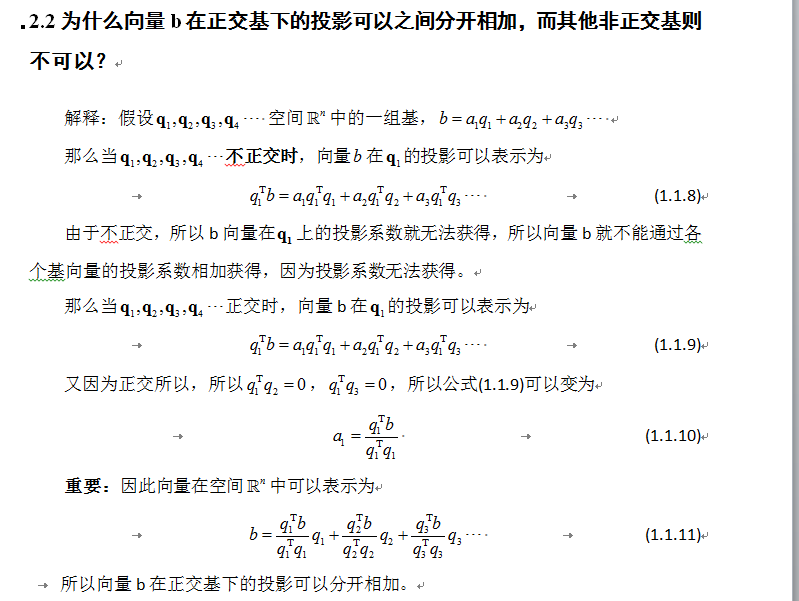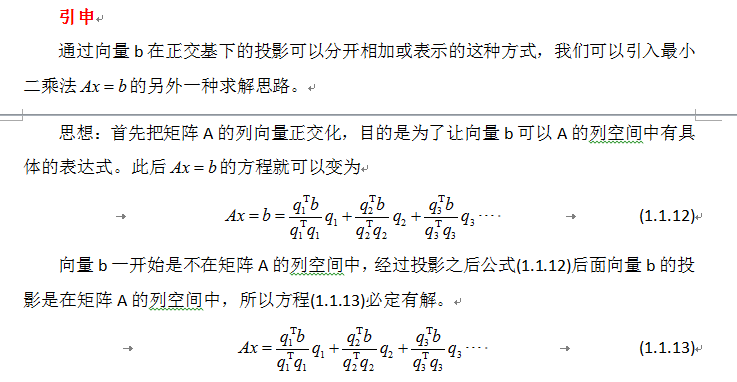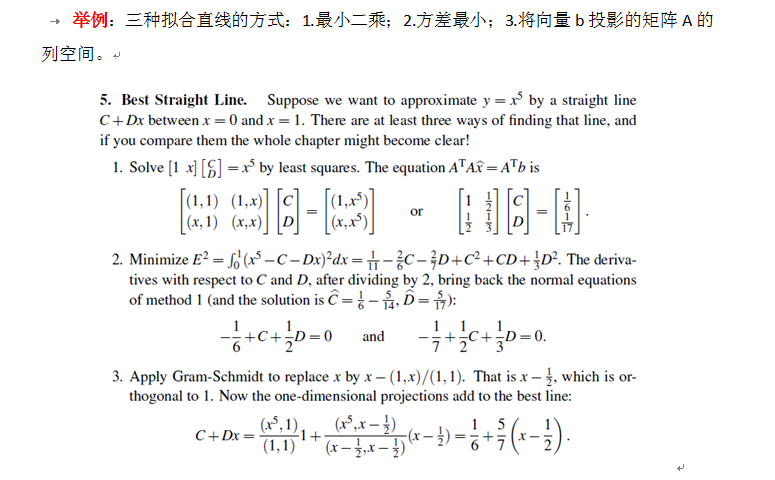展开全文• 正交（orthogonality）、正交集（orthogonal set）、单位正交集 定义：如果向量uuu和向量vvv是相互正交的，则有：u⋅v=0u\cdot v=0u⋅v=0。 因为对于零向量，都有0T⋅v=00^T\cdot v=00T⋅v=0，所以零向量与RnR^nRn中...线性代数 matlab
• ##标准正交基与坐标系（单位向量互相垂直构成坐标系） 任意两个二位向量，只要他们正交，长度为单位长度，就可构成一个标准正交基 三维中三个向量符合这个条件也构成标准正交基 全局模型储存在的坐标系：全局坐标系...算法
• 正交基和格拉姆-施密特正交化假设我们有是是三个无关向量a,b,ca,b,c，如果他们是正交的，那么会多问题都变得容易了。例如要计算vv到第一个向量的投影，只需计算(aTv)a(a^Tv)a，投影到前两个向量所在的平面只需相加...傅里叶级数 QR分解
• 长此以往，我们的大脑习惯了标准正交基上建立的直观，因此我们自己分析问题时，有时尽管不涉及长度和角度概念，我们也往往自然而然的建立标准正交基下的坐标系，从而进一步固化加强我们对标准正交基的直观和依赖。...
• title: 【线性代数】4-4:正交基和Gram算法(Orthogonal Bases and Gram-Schmidt) categories: Mathematic Linear Algebra keywords: Orthogonal Matrix Q Gram-Schmidt Algorithm QR toc: true date: 2017...
• 平面上线性变换与形变线性变换坐标的旋转变换正体字母变换为斜体字母刚体运动的矩阵齐次坐标系中的平移矩阵旋转实例向量改变后坐标值的变化正交坐标系固定坐标系和载体坐标系以数据为基础建立坐标系三级目录 ...线性代数
• 大部分人在考研时候都是直接背下来这个正交化过程对吧，或者也根本没有搞懂为啥这样操作就能够得到正交化的，现在就结合我的理解来分析一下这个原理吧 1.首先我们看看这个正交化过程，因为a1,a2...an为一组向量...线性代数 正交化 基向量
• 正交基和标准正交基： 前言： 经过上一次线性代数学习之向量空间，维度，和四大子空间的学习，对于空间的概念已经有了非常深刻的认识了，而描述空间很重要的方式除了维度以外，那就是空间的基了，而如小标题所示...线性代数 机器学习
• 基是无关向量的集合，他们可以生成一个空间。...正交基的想法是线性代数基本内容之一，我们需要一个基将几何转变为代数计算，而正交基可以让计算更加容易，再特殊一点：向量长度为1。对于一个正交基(正交单位向量)，子空间
• 文章目录第三章 正交1. 正交向量与子空间1.1. 内积1.2. 矩阵四大子空间的正交关系1.3. 矩阵的真正作用2.... 正交基，Gram-Schmidt正交化法4.1. 正交基4.1.1. 正交矩阵4.1.2. 正交变换4.1.3. 正交基4.2. Sch...线性代数 矩阵论 最小二乘法
• ## 正交矩阵

千次阅读 2019-02-23 15:37:23
• 施密特正交化（Schmidt orthogonalization）是求欧氏空间正交基的一种方法。从欧氏空间任意线性无关的向量组α1，α2，……，αm出发，求得正交向量组β1，β2，……，βm，使由α1，α2，……，αm与向量组β1，β2...
• ▼3.3 施密特正交化：寻找最佳投影在本章的前面两个小节里，我们通过向指定子空间进行投影，探索到了如何寻找“最近距离”的有效途径，通过理论分析和推导，成功得出了一组描述投影向量p和投影矩阵P的计算公式...
• ## 正交向量 正交矩阵

千次阅读 2017-11-12 22:36:10
• 2 向量的正交 3 函数的正交 4 函数正交的意义 1 向量内积和外积 1.1 向量内积(点乘) 假设 a = [a1,a2,...,an], b = [b1,b2,...,bn]； 则a与b的内积为 a·b = a1·b1+a2·b2+...+a3·b3 = |a||b|cosθ； ...
• 由于平面波具有标准正交化和能量单一性的特点,对任何原子都适用且等同对待空间中的任何区域,不需要修正重叠误差。因此平面波函数基组适合许多体系,其简单性使之成为求解Kohn-Sham方程的高效方案之一。另外,赝势的...
• 同时任何图像都可以分解为图像之和，基图像之间是相互正交的，图像正交变换的本质就是寻找合适的图像来表达图像。图像的正交变换一般分为三大类：正弦余弦型变换方波型变换基于特征向量的变换冲激函数通常也称...数字图像处理 离散线性变换
• 正交向量与正交化线性相关正交向量基正交化 线性相关 定义 定义1：在向量空间 VVV 的一组向量 AAA ：α1,α2,⋯αm\alpha_{1},\alpha_{2},\cdots \alpha_{m}α1​,α2​,⋯αm​，如果存在不全为零的数 k1,k2,⋯...线性代数 矩阵
• 这部分我们有两个目标。一是了解正交性是怎么让 x^\hat xx^ 、ppp ...1. 标准正交基 向量 q1,⋯&amp;ThinSpace;,qnq_1, \cdots, q_nq1​,⋯,qn​ 是标准正交的，如果它们满足如下条件： qiTqj={0，if&nbsp;i...
• 根据第一步得到的三个（单位向量表示的）坐标轴，这个就是平面旋转变换的正交基。逆变换后作用于平面，就可以把平面旋转到基坐标系。 如果是对平面形式的点云变换，只需要用求出来的变换作用于点云即可。 结果 ...
• 平面内每条直线是子空间，两直线垂直时，是平面正交分解。三维空间中，平面和直线垂直时是正交分解。几何上是如何定义直线垂直平面的？直线垂直于平面内任意直线，据此定义子空间垂直。 定义 子空间正交 两个子...线性代数 矩阵
• 我们在高中就知道，两个平面向量正交的时候时垂直的，写成向量乘法就是。在学习了线性代数后，我们把它写成了。这里的向量可以是任意维数的，比如。上面的点乘被称为求取向量的内积，即对应元素的求积累加。那么，......